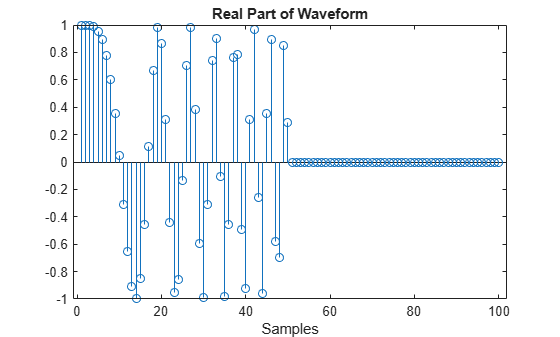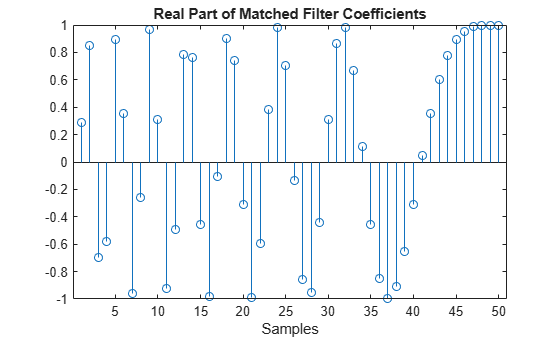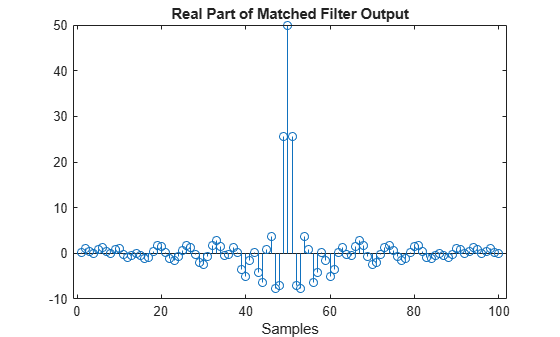# step

System object: phased.MatchedFilter
Package: phased

Perform matched filtering

## Syntax

```Y = step(H,X) Y = step(H,X,COEFF) [Y,GAIN] = step(___) ```

## Description

### Note

Starting in R2016b, instead of using the `step` method to perform the operation defined by the System object™, you can call the object with arguments, as if it were a function. For example, ```y = step(obj,x)``` and `y = obj(x)` perform equivalent operations.

`Y = step(H,X)` applies the matched filtering to the input `X` and returns the filtered result in `Y`. The filter is applied along the first dimension. `Y` and `X` have the same dimensions. The initial transient is removed from the filtered result.

The size of the first dimension of the input matrix can vary to simulate a changing signal length. A size change can occur, for example, in the case of a pulse waveform with variable pulse repetition frequency.

`Y = step(H,X,COEFF)` uses the input `COEFF` as the matched filter coefficients. This syntax is available when you set the `CoefficientsSource` property to ```'Input port'```.

`[Y,GAIN] = step(___)` returns additional output `GAIN` as the gain (in decibels) of the matched filter. This syntax is available when you set the `GainOutputPort` property to `true`.

### Note

The object performs an initialization the first time the object is executed. This initialization locks nontunable properties (MATLAB) and input specifications, such as dimensions, complexity, and data type of the input data. If you change a nontunable property or an input specification, the System object issues an error. To change nontunable properties or inputs, you must first call the `release` method to unlock the object.

## Examples

expand all

Construct a linear FM waveform with a sweep bandwidth of 300 kHz and a pulse width of 50 μs. Obtain the matched filter coefficients using the `getMatchedFilter` method. Then, use the `step` to match-filter the waveform.

```waveform = phased.LinearFMWaveform('SweepBandwidth',3e5,... 'OutputFormat','Pulses','SampleRate',1e6,... 'PulseWidth',50e-6,'PRF',1e4); wav = waveform();```

Plot the entire waveform. The length of the waveform is the pulse repetition interval (100 samples).

```stem(real(wav)) xlabel('Samples') title('Real Part of Waveform')```Obtain the matched filter coefficients for the linear FM waveform. The length of the matched filter coefficients is the length of the pulse.

```mfcoeffs = getMatchedFilter(waveform); stem(real(mfcoeffs)) xlabel('Samples') title('Real Part of Matched Filter Coefficients')```Use `phased.MatchedFilter` `step` method to obtain the matched filter output.

```filter = phased.MatchedFilter('Coefficients',mfcoeffs); mfoutput = filter(wav); stem(real(mfoutput)) xlabel('Samples') title('Real Part of Matched Filter Output')```